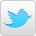#Scales

A scale is a sequence of notes starting from the root note and following a formula of tones and semi-tones to create the sound of the scale.
The Major scale is usually used as a starting point when learning music and scales. Its formula is:
Tone Tone Semitone T T T S we can see how this formula creates the notes of the scale if we follow the sequence from the note A:

 A B C# D E F# G# A T T S T T T S

The notes found in the key of A give us 3 accidentals C#, F# and G#. This is what you’ll find on music notation at the start of each line of a piece of music and is called a key signature ; this tells the player what key they are playing in and also which notes should be sharpened or flattened when playing the music. When trying to understand scales it’s a good idea to first of all play the notes on one string this lets you see the tone and semitone distance between the notes and hear the sound of the scale as you play it. Once you’ve done that you could try one octave of one pattern of the scale.
Tones and semitones are a good way of understanding the distance between notes in a scale but are less useful when comparing one scale to another, in this case we can use numbers to understand the difference between two scales. It should be noted that scales can only be compared in the same key i.e. the A Major Scale and the A Natural Minor Scale.
When using a numeric formula to understand a scale we can quickly see which notes have been altered from one scale to the next and also in which direction the note has moved – if it has been flattened or sharpened -. In the example below we will compare a Major scale with a Natural Minor scale in the key of C
First we assign numbers to the scale, as the Major scale is seen as the starting point its numbers are unaltered (no flats or sharps)

 C D E F G A B C 1 2 3 4 5 6 7 8

the minor scale has these notes:

 C D Eb F G Ab Bb C

so immediately we can see that 3 notes have been flattened: E the 3rd note; A the 6th note and B the 7th. Our numeric formula for the minor scale then would be:
 1 2 b3 4 5 b6 b7 8
(you’ll notice in the numeric formula the flat comes before the number not after because it instructs us to flatten the 3rd note whereas in the note name it tells us the E is already flattened).

You can work out the formulae for scales yourself, follow these steps
1. Play one octave of a known scale and write down the notes as you go (Make sure to double check the notes are right or your formula won’t be correct).
2. Play and write down the notes of the Major scale in the same key.
3. Compare the two sets of notes and alter the numbers of the notes which are different in your chosen scale. Simply add a flat b before the number if the note is lower than the Major scale or add a sharp # if the note is higher than the Major scale note. If the scale you’re working out doesn’t have that note at all simply miss the number out.
By doing this with all scales we can create a list of scale formulae which show us how scales compare.

### List of Scales

Scale Formula
Major 1 2 3 4 5 6 7 8
Natural Minor 1 2 b3 4 5 b6 b7 8
Harmonic Minor 1 2 b3 4 5 b6 7 8
Pentatonic Major 1 2 3   5 6   8
Pentatonic Minor 1   b3 4 5   b7 8
Blues 1   b3 4 b5 5   b7 8